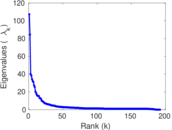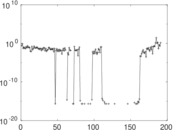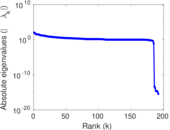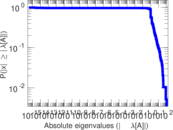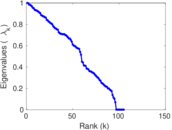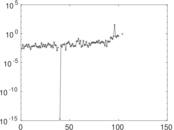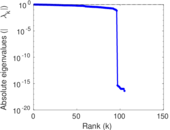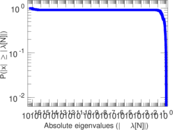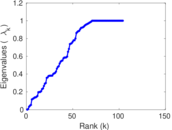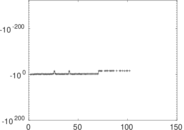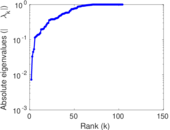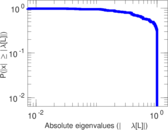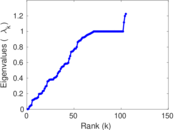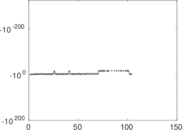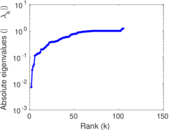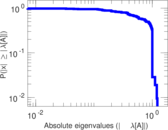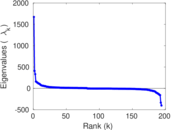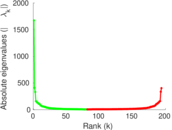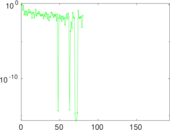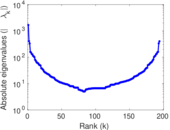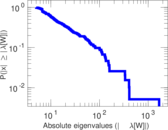# Wiktionary edits (qu)

This is the bipartite edit network of the Quechua Wiktionary. It contains users and pages from the Quechua Wiktionary, connected by edit events. Each edge represents an edit. The dataset includes the timestamp of each edit.

 Code `mqu` Internal name `edit-quwiktionary` Name Wiktionary edits (qu) Data source http://dumps.wikimedia.org/ AvailabilityDataset is available for download Consistency checkDataset passed all tests Category Authorship network Dataset timestamp 2017-10-20 Node meaning User, article Edge meaning Edit Network formatBipartite, undirected Edge typeUnweighted, multiple edges Temporal dataEdges are annotated with timestamps

## Statistics

 Size n = 1,689 Left size n1 = 194 Right size n2 = 1,495 Volume m = 6,491 Unique edge count m̿ = 3,686 Wedge count s = 391,396 Claw count z = 40,526,033 Cross count x = 3,770,614,368 Square count q = 314,130 4-Tour count T4 = 4,086,320 Maximum degree dmax = 918 Maximum left degree d1max = 918 Maximum right degree d2max = 74 Average degree d = 7.686 20 Average left degree d1 = 33.458 8 Average right degree d2 = 4.341 81 Fill p = 0.012 709 0 Average edge multiplicity m̃ = 1.760 99 Size of LCC N = 1,443 Diameter δ = 14 50-Percentile effective diameter δ0.5 = 3.758 69 90-Percentile effective diameter δ0.9 = 7.689 57 Median distance δM = 4 Mean distance δm = 4.858 03 Gini coefficient G = 0.771 466 Balanced inequality ratio P = 0.186 258 Left balanced inequality ratio P1 = 0.106 763 Right balanced inequality ratio P2 = 0.253 428 Relative edge distribution entropy Her = 0.787 337 Power law exponent γ = 2.667 64 Tail power law exponent γt = 1.971 00 Tail power law exponent with p γ3 = 1.971 00 p-value p = 0.000 00 Left tail power law exponent with p γ3,1 = 1.661 00 Left p-value p1 = 0.014 000 0 Right tail power law exponent with p γ3,2 = 2.031 00 Right p-value p2 = 0.000 00 Degree assortativity ρ = −0.114 878 Degree assortativity p-value pρ = 2.646 54 × 10−12 Spectral norm α = 107.211 Algebraic connectivity a = 0.007 272 33 Spectral separation |λ1[A] / λ2[A]| = 1.270 36 Controllability C = 1,310 Relative controllability Cr = 0.780 691

## Plots

### Fruchterman–Reingold graph drawing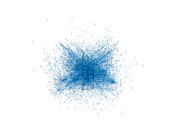### Degree distribution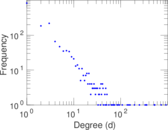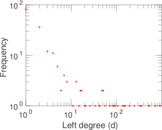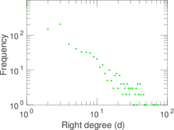### Cumulative degree distribution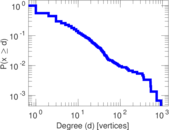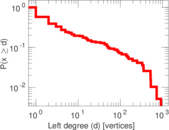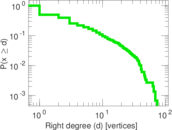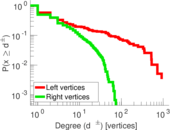### Lorenz curve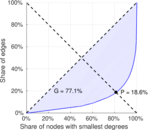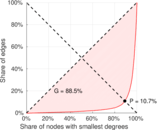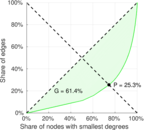### Spectral distribution of the adjacency matrix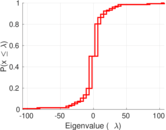### Spectral distribution of the normalized adjacency matrix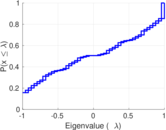### Spectral distribution of the Laplacian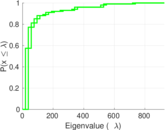### Spectral graph drawing based on the adjacency matrix### Spectral graph drawing based on the Laplacian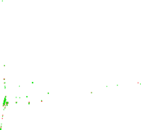### Spectral graph drawing based on the normalized adjacency matrix### Degree assortativity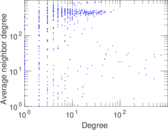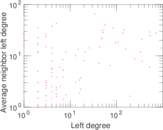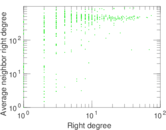### Zipf plot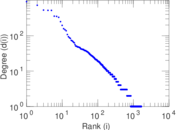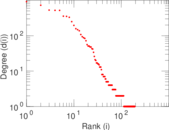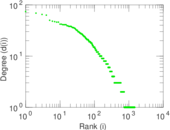### Hop distribution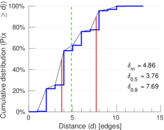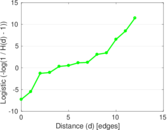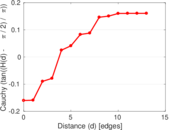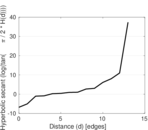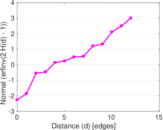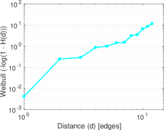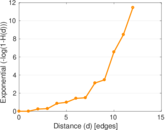### Double Laplacian graph drawing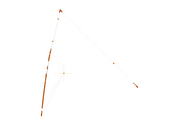### Delaunay graph drawing### Edge weight/multiplicity distribution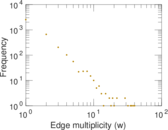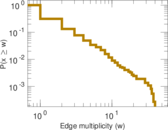### Temporal distribution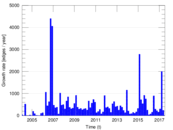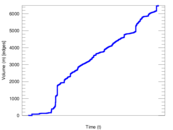### Temporal hop distribution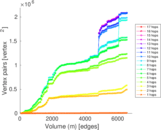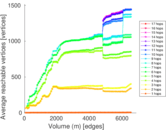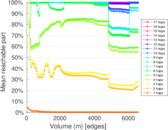### Diameter/density evolution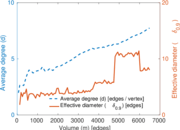### Matrix decompositions plots